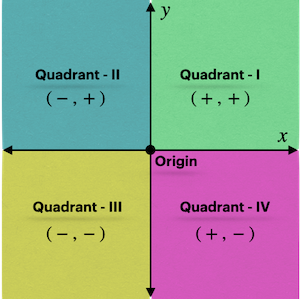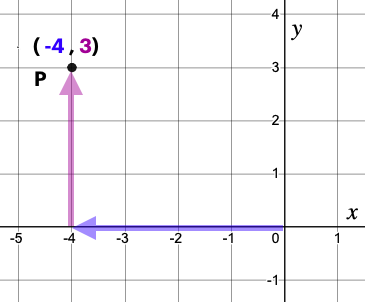What your children are learning in 6th grade math might have changed since you were in school–but not to worry, we’ve got you covered. Inside this article you will find a complete list of all the topics covered in 6th grade math, as well as definitions and examples of each topic. Knowing this, you will be able to communicate with your kids about their math homework. These general topics are the standard cirriculum in 6th grade math across most of the U.S.

## Adding, Subtracting, Multiplying and Dividing Integers

Integers are a set of whole numbers (0, 1, 2, 3, ....) and their opposites. For example, 1, -1,  2, -2, 3, -3, and so on. Students will learn how to add, subtract, multiply and divide numbers which are both positive and negative in 6th grade math.

## Prime Factorization

Factors are numbers which, multiplied together make up another number. For example 3 and 4 are factors of 12, since 3 times 4 equals 12. Prime factorization is finding the prime numbers that multiply to make the original number. A prime number is greater than 1 and has just two factors, itself and the number 1. Here is a list of prime numbers from 1-100:  2, 3, 5, 7, 11, 13, 17, 19, 23, 29, 31, 37, 41, 43, 47, 53, 59, 61, 67, 71, 73, 79, 83, 89, and 97.

Example 1: Find the prime factorization of 56.

Solution:  2×2×2×7

## Greatest Common Factor (GCF) and Least Common Multiple (LCM)

GCF is the largest common factor of all the possible factors of the given numbers.

LCM is the smallest common multiple of the given numbers. This skill will be used to solve a variety of math problems going forward in math.

## Working With Fractions

A fraction represents a relation of a part(s) to the whole, where the whole is divided into equal parts. In 6th grade, students learn how to add, subtract, multiply and divide fractions. Students will also learn how to convert fractions to decimals i.e. ¾ is equal to 75%.

In 6th grade math students also learn how to compare fractions, finding which of two fractions are greater than the other.

## Order of Operations

When solving a math expression, order of operations is used as a rule to perform the sequence of steps to solve the equation. These are the basic rules which students will use to solve math equations.

## Unit Rate

Unit rate is used to find the rate of something, for example how fast is a car going which travels 100 miles in 2 hours. The answer would be the unit rate of 50 miles per hour.

## Algebraic Expressions & Properties

An expression is a mathematical statement comprising variables (X Y Z etc.), numbers, and operators (multiplication, division etc.) An expression can be of two types–numerical expression and algebraic expression. A numerical expression involves only numbers and mathematical operators (no variables). An algebraic expression involves numbers, variables, and mathematical operators.

## Introductions to Equations

An equation is a mathematical statement that shows that two expressions are equal. In 6th grade math students get an introduction to solving simple equations.

## Coordinate Planes

The coordinate plane is a 2-D surface divided into 4 quadrants by two intersecting lines called the X-axis and Y- axis.In 6th grade math students get an introduction to graphing points on a coordinate plane.## Statistics–Mean, Median, Mode & Range

Statistics is a scientific method that uses mathematics to collect data and information for a particular purpose (survey). In 6th grade math students get an introduction to statistics and its basic terms. The mean is the most widely used measure of central tendency in which the average of the data is taken. The median is a measure of central tendency where the mid-value of the data is determined. Mode is the number that is repeated most frequently in a data set. Range is the greatest value in the data set minus the least value in the data set.

## How MathPrep Can Help

MathPrep is the ultimate math practice site. Our goal is to help children become confident math students and eliminate all math test anxiety forever. We do this by giving students what they need most to excel at math–practice.

MathPrep has thousands of practice problems and gives students the ability to take timed practice tests so they can be 100% prepared for their math tests. Our software tracks students progress and guides students to better abilities on a gradient of difficulty using cutting edge artificial intelligence.